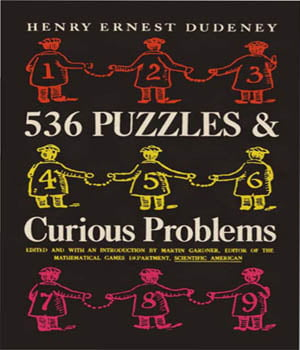# 536 Puzzle and Curious Problems

536 Puzzle and Curious Problems Henry Ernest Dudeney (the last name is pronounced with a long “u” and a strong accent on the first syllable, as in “scrutiny”) was England’s greatest maker of puzzles. With respect to mathematical puzzles, especially problems of more than trivial mathematical interest, the quantity and quality of his output surpassed that of any other puzzlist before or since, in or out of England.

Dudeney was born at Mayfield, in Sussex, on April 10, 1857, the son of a local schoolmaster. His father’s father, John Dudeney, was well known in Sussex as a shepherd who had taught himself mathematics and astronomy while tending sheep on the downs above Lewes, a town fifty miles south of London. Later he became a schoolmaster in Lewes. Henry Dudeney, himself a self-taught mathematician who never went to college, was understandably proud to be the grandson of this famous shepherd-mathematician.536 Puzzle and Curious Problems Henry Ernest Dudeney (the last name is pronounced with a long “u” and a strong accent on the first syllable, as in “scrutiny”) was England’s greatest maker of puzzles. With respect to mathematical puzzles, especially problems of more than trivial mathematical interest, the quantity and quality of his output surpassed that of any other puzzlist before or since, in or out of England.

## Content

ARITHMETICAL AND ALGEBRAICAL PROBLEMS

• Money Puzzles
• Age Puzzles
• Clock Puzzles
• Speed and Distance Puzzles
• Weight Puzzles
• Digital Puzzles
• Skeleton Puzzles
• Cryptarithm Puzzles
• Miscellaneous Puzzles

GEOMETRICAL PROBLEMS

• Triangle, Square, and other Polygon Puzzles
• Circle Puzzles
• Dividing-the-Plane Puzzles
• Plane Geometry Puzzles
• Solid Geometry Puzzles
• Dissection Puzzles
• Paper Folding Puzzles
• Moving Counter Puzzles

COMBINATORIAL AND TOPOLOGICAL PROBLEMS

• Magic Square Puzzles
• Magic Star Puzzles
• Liquid Pouring Puzzles
• Route and Network Puzzles
• Point Alignment Puzzles
• Map Coloring Puzzles
• Miscellaneous Combinatorial Puzzles

GAME PUZZLES
DOMINO PUZZLES
MATCH PUZZLES
UNCLASSIFIED PUZZLES